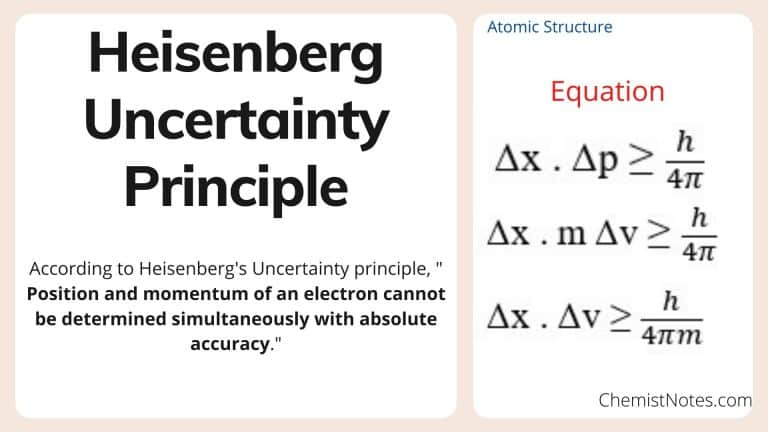# Heisenberg Uncertainty Principle Definition, Equation, and Application

Heisenberg Uncertainty principle was proposed by a German physicist, Werner Heisenberg in 1927, also known as the Uncertainty principle. This principle states that it is impossible to measure both the velocity and position of a particle accurately at the same time.

## Heisenberg Uncertainty Principle Definition

According to this principle, ” Position and momentum of an electron cannot be determined simultaneously with absolute accuracy.” This principle arises from the duality wave nature of particles. Since electron, a particle that is too small to observe must be disturbed to measure the parameters. On disturbing electron either by applying electric or magnetic force, it either changes its velocity or position.

In other words, the particle with a well-defined wavelength while moving has precise velocity, but the position of particle becomes uncertain. On the other hand, the particle having not defined wavelength have a precise position but with uncertainty in its position. Hence, It is very tough to measure both quantities accurately at the same time.

Thus, the more accuracy we calculate in the particle’s position, the less the precision will be in its velocity, and vice-versa.

## Heisenberg Uncertainty Principle equation

This is the mathematical formula or equation which clears that the accuracy in the measurement of one quantity (say velocity) causes uncertainty/error in other quantity (momentum), and vice-versa).On a concluding note, Heisenberg’s Uncertainty principle tells us that it is impossible to know both the position and velocity exactly at the same time.

## Application of Heisenberg Uncertainty Principle

Some of the major applications of this uncertainty principle are:

1. Non-existence of free electrons in the nucleus
2. Width of spectral lines

## Limitation of Heisenberg Uncertainty Principle

• Only valid for dual-nature small particles like atom and sub-atomic particles

## FAQs

### Explain Heisenberg Uncertainty Principle

According to Heisenberg’s Uncertainty principle, ” Position and momentum of an electron cannot be determined simultaneously with absolute accuracy.